# ILP Part 82 — S and P

This is the eightieth second part of the ILP series. For your convenience you can find other parts in the table of contents in Part 1 – Boolean algebra

Today we’re going to solve S and P. The idea is: we start with two non-negative integersand. We calculate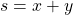and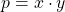. Now we take digits of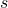and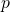and want all of them to be distinct and form a continuous sequence after sorting. For instance, for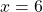and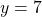we get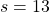and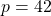so digits are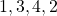which are distinct and form a proper sequence.

We want to find a solution for someindicating how long the sequence should be.

Solution:

We start with definition ofin line 1.

Next, we calculate how many digits there are in the sum and in result of multiplication. We assume the latter is bigger than the former.

Next, we define initial variables (lines 6-7) and calculations (9-10).

Next, we extract digits in base 10. We make sure that the result of decomposition is not too big (lines 17-20 and 29-32). We basically zero-out too big digits.

Next, we make sure that the decomposition is not too low. So the most significant digit must be non-zero (lines 21 and 33).

Finally, we take all digits and make sure they are distinct (line 39). Next, we calculate min and max (41-42) and then make sure that they differ by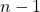. Since all digits are distinct, we get the required effect.

Output: